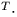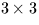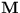Next: SLA_INTIN - Decode an Integer Number
Up: SUBPROGRAM SPECIFICATIONS
Previous: SLA_HFK5Z - Hipparcos to FK5, no P.M.

## SLA_IMXV - Apply 3D Reverse Rotation

ACTION:
Multiply a 3-vector by the inverse of a rotation matrix (single precision).

CALL:
CALL sla_IMXV (RM, VA, VB)

GIVEN:

 R(3,3) RM rotation matrix VA R(3) vector to be rotated

RETURNED:

 R(3) VB result vector

NOTES:
1.
This routine performs the operation:
b = Ma
where a and b are the 3-vectors VA and VB respectively, and M is thematrix RM.
2.
The main function of this routine is apply an inverse rotation; under these circumstances,is orthogonal, with its inverse the same as its transpose.
3.
To comply with the ANSI Fortran 77 standard, VA and VB must not be the same array. The routine is, in fact, coded so as to work properly on the VAX and many other systems even if this rule is violated, something that is not, however, recommended.Next: SLA_INTIN - Decode an Integer Number
Up: SUBPROGRAM SPECIFICATIONS
Previous: SLA_HFK5Z - Hipparcos to FK5, no P.M.

SLALIB --- Positional Astronomy Library